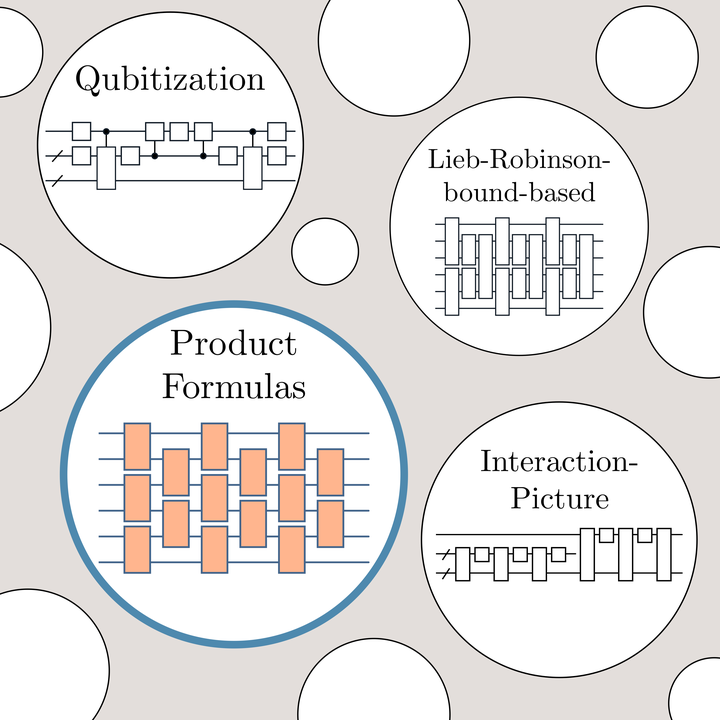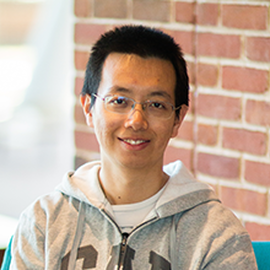# A Theory of Trotter ErrorImage credit: Minh C. Tran

### Abstract

The Lie-Trotter formula, together with its higher-order generalizations, provides a simple approach to decomposing the exponential of a sum of operators. Despite significant effort, the error scaling of such product formulas remains poorly understood.

We develop a theory of Trotter error that overcomes the limitations of prior approaches based on truncating the Baker-Campbell-Hausdorff expansion. Our analysis directly exploits the commutativity of operator summands, producing tighter error bounds for both real- and imaginary-time evolutions. Whereas previous work achieves similar goals for systems with geometric locality or Lie-algebraic structure, our approach holds in general.

We give a host of improved algorithms for digital quantum simulation and quantum Monte Carlo methods, nearly matching or even outperforming the best previous results. Our applications include: (i) a simulation of second-quantized plane-wave electronic structure, nearly matching the interaction-picture algorithm of Low and Wiebe; (ii) a simulation of $k$-local Hamiltonians almost with induced $1$-norm scaling, faster than the qubitization algorithm of Low and Chuang; (iii) a simulation of rapidly decaying power-law interactions, outperforming the Lieb-Robinson-based approach of Tran et al.; (iv) a hybrid simulation of clustered Hamiltonians, dramatically improving the result of Peng, Harrow, Ozols, and Wu; and (v) quantum Monte Carlo simulations of the transverse field Ising model and quantum ferromagnets, tightening previous analyses of Bravyi and Gosset.

We obtain further speedups using the fact that product formulas can preserve the locality of the simulated system. Specifically, we show that local observables can be simulated with complexity independent of the system size for power-law interacting systems, which implies a Lieb-Robinson bound nearly matching a recent result of Tran et al.

Our analysis reproduces known tight bounds for first- and second-order formulas. We further investigate the tightness of our bounds for higher-order formulas. For quantum simulation of a one-dimensional Heisenberg model with an even-odd ordering of terms, our result overestimates the complexity by only a factor of $5$. Our bound is also close to tight for power-law interactions and other orderings of terms. This suggests that our theory can accurately characterize Trotter error in terms of both the asymptotic scaling and the constant prefactor.

Type
Publication
Phys. Rev. X 11, 011020##### Yuan Su
###### Research Scientist

I work on quantum algorithms for simulating Hamiltonian dynamics. I am particularly interested in the design, analysis, implementation, and application of quantum simulation.